# Selina Solutions Concise Maths Class 10 Chapter 14 Equation of a Line Exercise 14(C)

Equation of a line can be expressed in various forms. All the types are discussed and examined in this exercise. Furthermore, the concept of equally inclined lines is also studied. Selina Solutions for Class 10 Maths help students boost their exam preparations and clear doubts on solving complex problems at the same time. Students can access Selina Solutions Concise Maths Class 10 Chapter 14 Equation of a Line Exercise 14(C) PDF, from the links given below.

## Selina Solutions Concise Maths Class 10 Chapter 14 Equation of a Line Exercise 14(C) Download PDF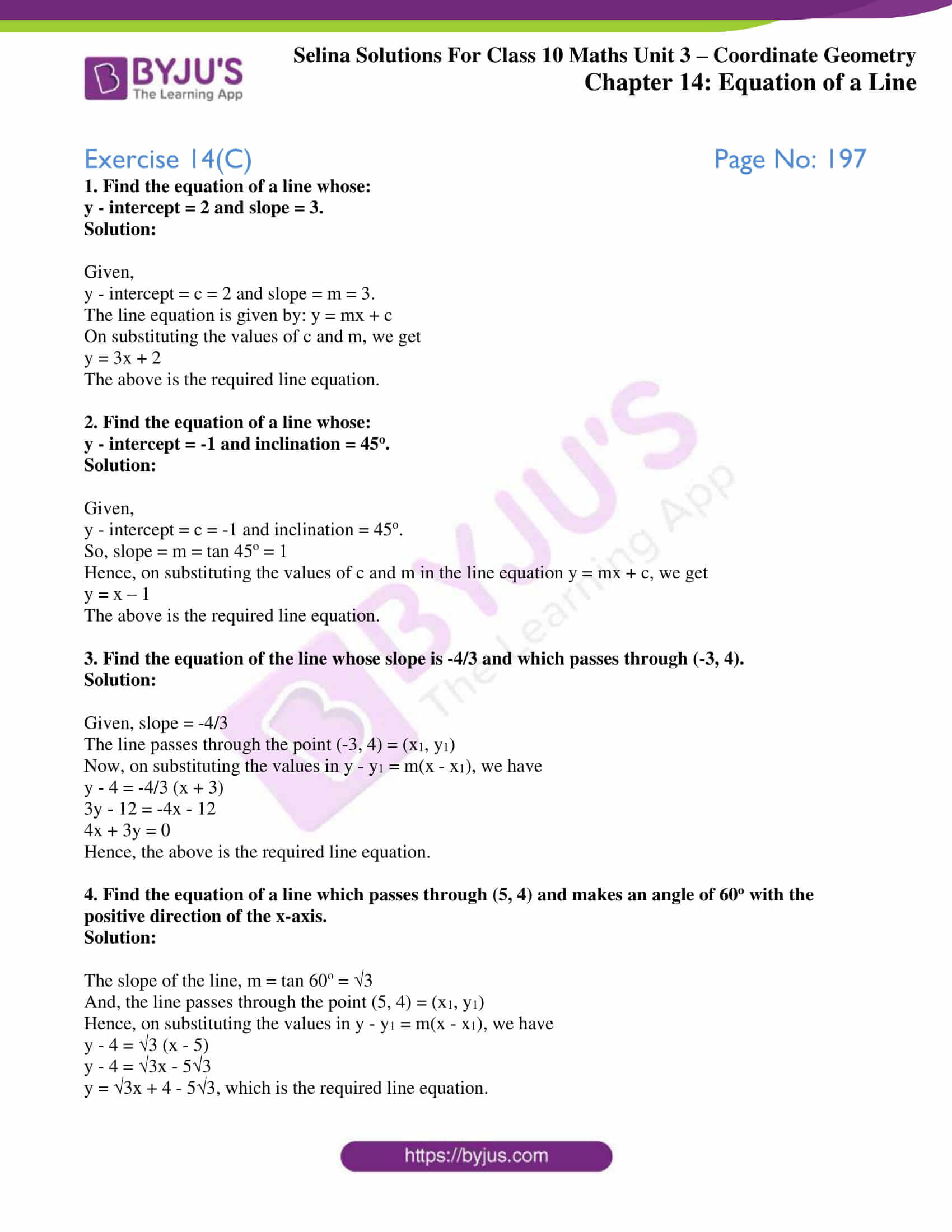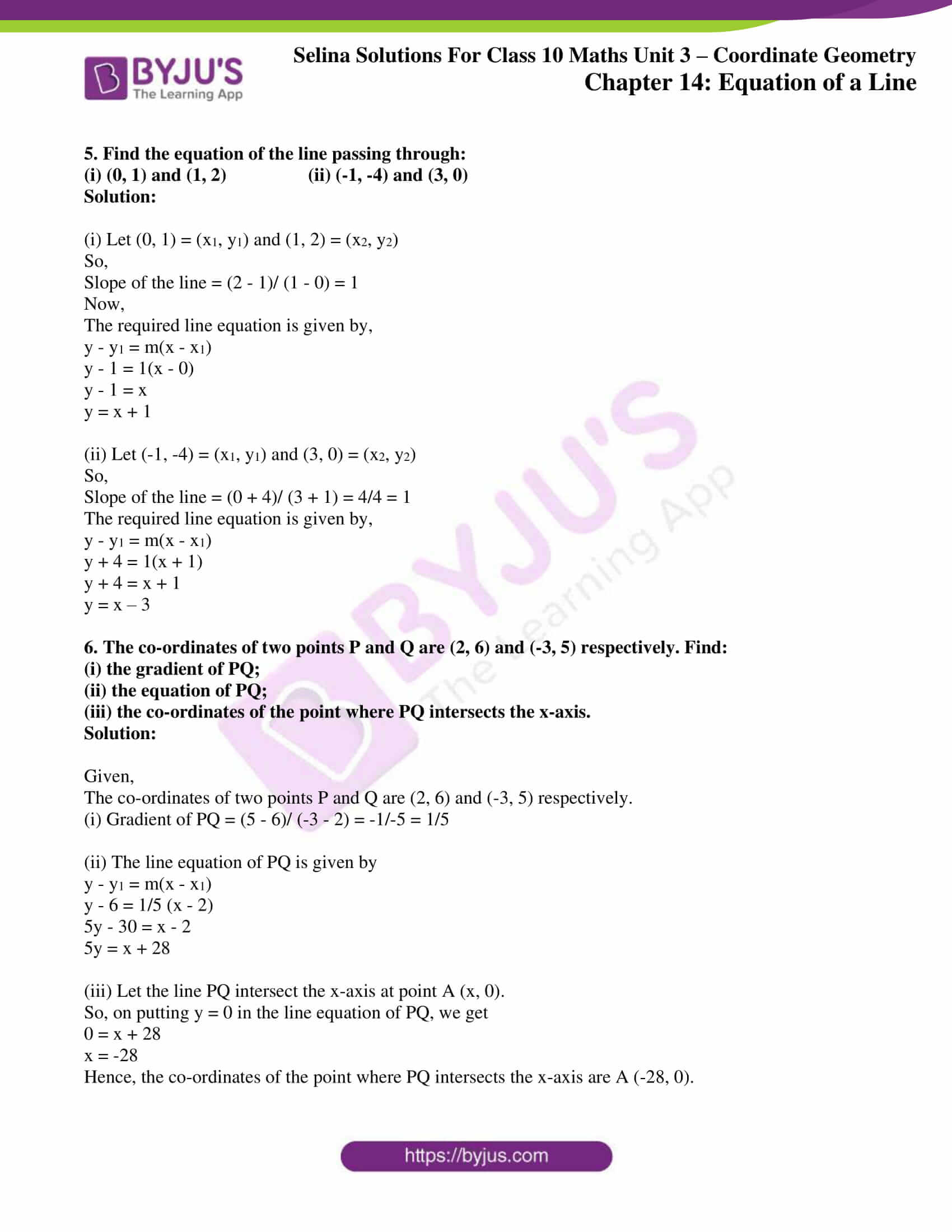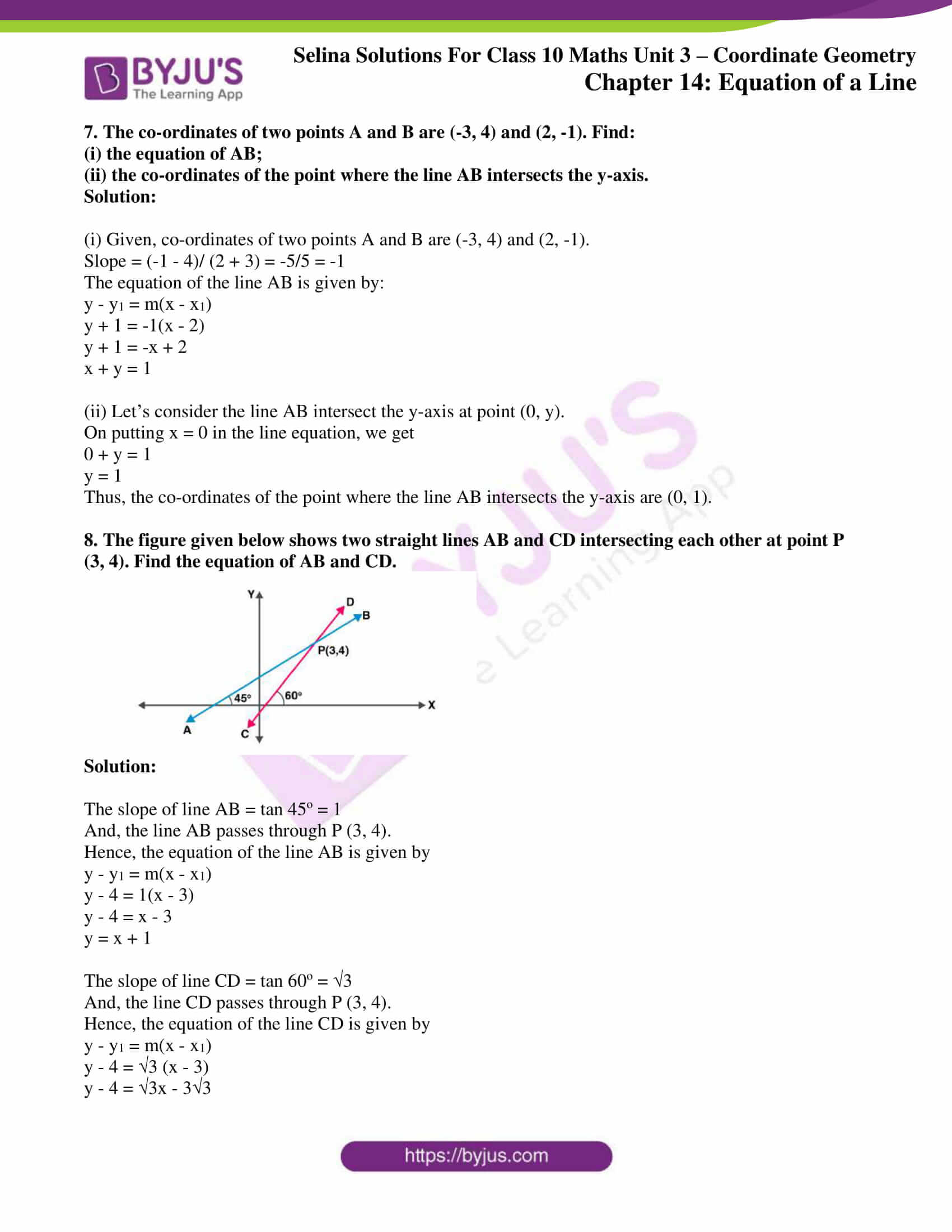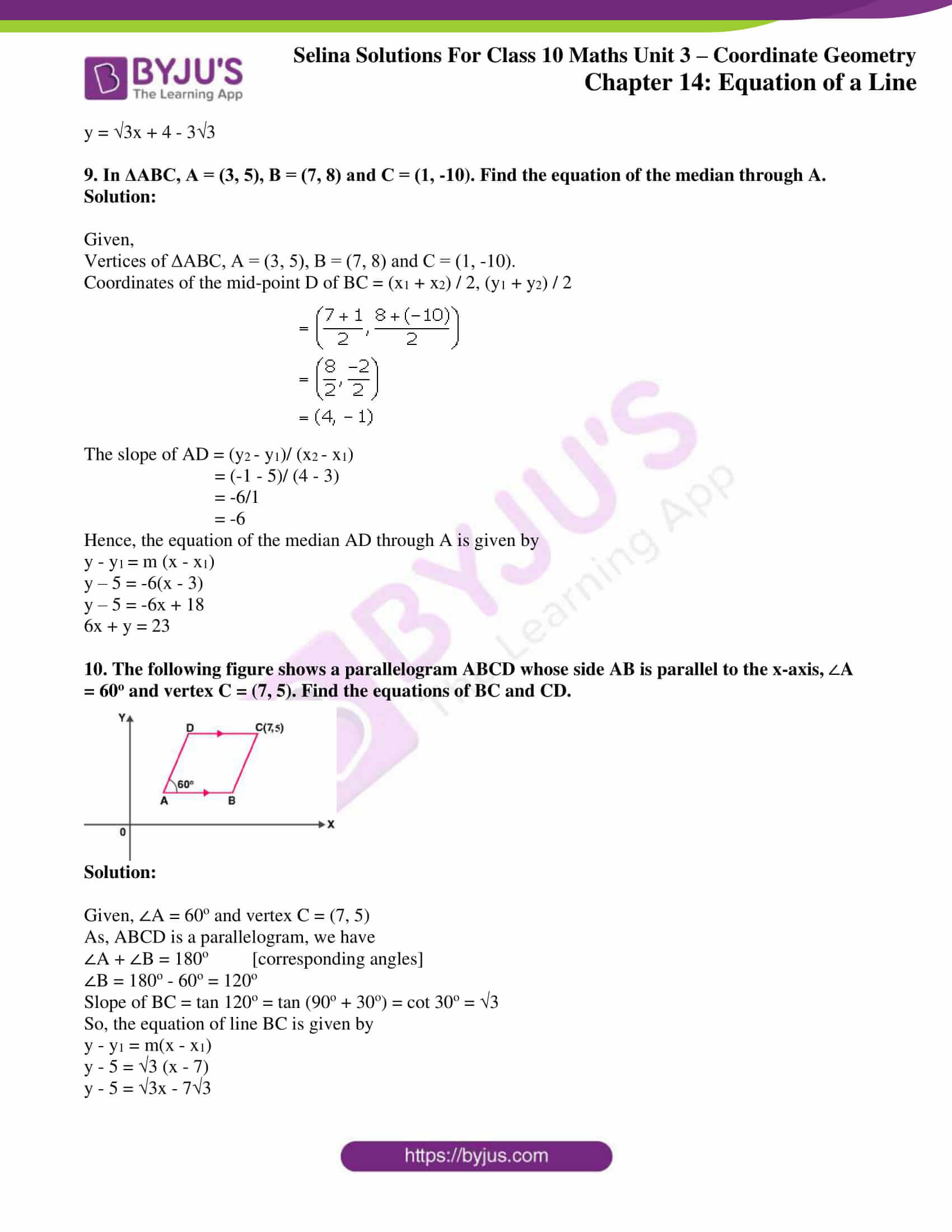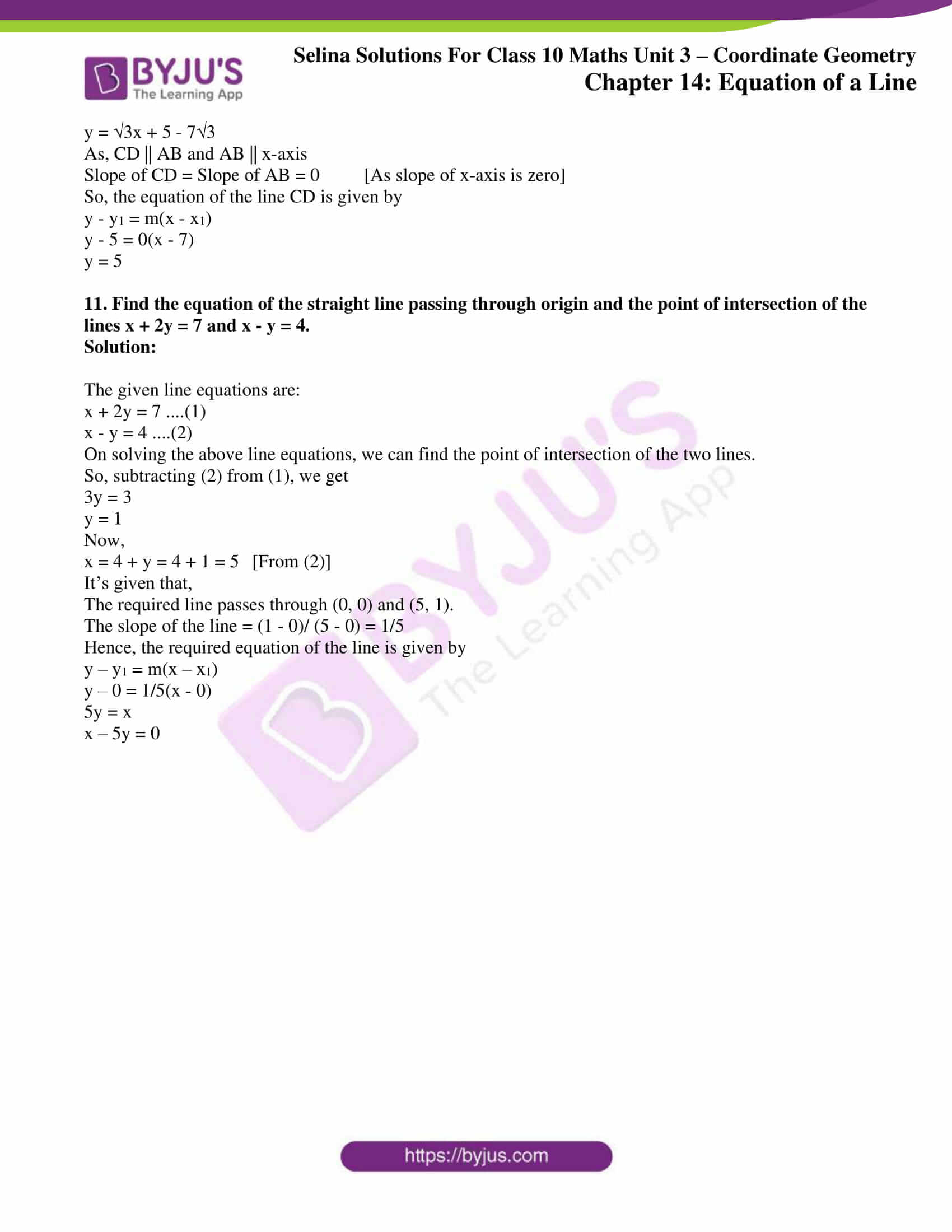### Access other exercises of Selina Solutions Concise Maths Class 10 Chapter 14 Equation of a Line

Exercise 14(A) Solutions

Exercise 14(B) Solutions

Exercise 14(D) Solutions

Exercise 14(E) Solutions

### Access Selina Solutions Concise Maths Class 10 Chapter 14 Equation of a Line Exercise 14(C)

#### Exercise 14(C) Page No: 197

1. Find the equation of a line whose:

y – intercept = 2 and slope = 3.

Solution:

Given,

y – intercept = c = 2 and slope = m = 3.

The line equation is given by: y = mx + c

On substituting the values of c and m, we get

y = 3x + 2

The above is the required line equation.

2. Find the equation of a line whose:

y – intercept = -1 and inclination = 45o.

Solution:

Given,

y – intercept = c = -1 and inclination = 45o.

So, slope = m = tan 45o = 1

Hence, on substituting the values of c and m in the line equation y = mx + c, we get

y = x – 1

The above is the required line equation.

3. Find the equation of the line whose slope is -4/3 and which passes through (-3, 4).

Solution:

Given, slope = -4/3

The line passes through the point (-3, 4) = (x1, y1)

Now, on substituting the values in y – y1 = m(x – x1), we have

y – 4 = -4/3 (x + 3)

3y – 12 = -4x – 12

4x + 3y = 0

Hence, the above is the required line equation.

4. Find the equation of a line which passes through (5, 4) and makes an angle of 60o with the positive direction of the x-axis.

Solution:

The slope of the line, m = tan 60o = √3

And, the line passes through the point (5, 4) = (x1, y1)

Hence, on substituting the values in y – y1 = m(x – x1), we have

y – 4 = √3 (x – 5)

y – 4 = √3x – 5√3

y = √3x + 4 – 5√3, which is the required line equation.

5. Find the equation of the line passing through:

(i) (0, 1) and (1, 2) (ii) (-1, -4) and (3, 0)

Solution:

(i) Let (0, 1) = (x1, y1) and (1, 2) = (x2, y2)

So,

Slope of the line = (2 – 1)/ (1 – 0) = 1

Now,

The required line equation is given by,

y – y1 = m(x – x1)

y – 1 = 1(x – 0)

y – 1 = x

y = x + 1

(ii) Let (-1, -4) = (x1, y1) and (3, 0) = (x2, y2)

So,

Slope of the line = (0 + 4)/ (3 + 1) = 4/4 = 1

The required line equation is given by,

y – y1 = m(x – x1)

y + 4 = 1(x + 1)

y + 4 = x + 1

y = x – 3

6. The co-ordinates of two points P and Q are (2, 6) and (-3, 5) respectively. Find:

(ii) the equation of PQ;

(iii) the co-ordinates of the point where PQ intersects the x-axis.

Solution:

Given,

The co-ordinates of two points P and Q are (2, 6) and (-3, 5) respectively.

(i) Gradient of PQ = (5 – 6)/ (-3 – 2) = -1/-5 = 1/5

(ii) The line equation of PQ is given by

y – y1 = m(x – x1)

y – 6 = 1/5 (x – 2)

5y – 30 = x – 2

5y = x + 28

(iii) Let the line PQ intersect the x-axis at point A (x, 0).

So, on putting y = 0 in the line equation of PQ, we get

0 = x + 28

x = -28

Hence, the co-ordinates of the point where PQ intersects the x-axis are A (-28, 0).

7. The co-ordinates of two points A and B are (-3, 4) and (2, -1). Find:

(i) the equation of AB;

(ii) the co-ordinates of the point where the line AB intersects the y-axis.

Solution:

(i) Given, co-ordinates of two points A and B are (-3, 4) and (2, -1).

Slope = (-1 – 4)/ (2 + 3) = -5/5 = -1

The equation of the line AB is given by:

y – y1 = m(x – x1)

y + 1 = -1(x – 2)

y + 1 = -x + 2

x + y = 1

(ii) Let’s consider the line AB intersect the y-axis at point (0, y).

On putting x = 0 in the line equation, we get

0 + y = 1

y = 1

Thus, the co-ordinates of the point where the line AB intersects the y-axis are (0, 1).

8. The figure given below shows two straight lines AB and CD intersecting each other at point P (3, 4). Find the equation of AB and CD.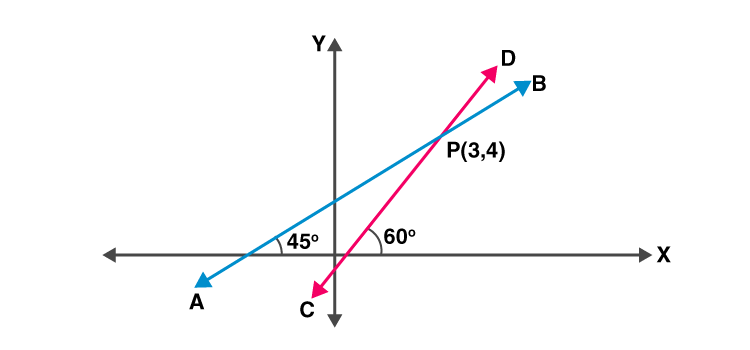Solution:

The slope of line AB = tan 45o = 1

And, the line AB passes through P (3, 4).

Hence, the equation of the line AB is given by

y – y1 = m(x – x1)

y – 4 = 1(x – 3)

y – 4 = x – 3

y = x + 1

The slope of line CD = tan 60o = √3

And, the line CD passes through P (3, 4).

Hence, the equation of the line CD is given by

y – y1 = m(x – x1)

y – 4 = √3 (x – 3)

y – 4 = √3x – 3√3

y = √3x + 4 – 3√3

9. In ΔABC, A = (3, 5), B = (7, 8) and C = (1, -10). Find the equation of the median through A.

Solution:

Given,

Vertices of ΔABC, A = (3, 5), B = (7, 8) and C = (1, -10).

Coordinates of the mid-point D of BC = (x1 + x2) / 2, (y1 + y2) / 2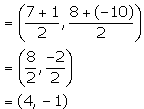The slope of AD = (y2 – y1)/ (x2 – x1)

= (-1 – 5)/ (4 – 3)

= -6/1

= -6

Hence, the equation of the median AD through A is given by

y – y1 = m (x – x1)

y – 5 = -6(x – 3)

y – 5 = -6x + 18

6x + y = 23

10. The following figure shows a parallelogram ABCD whose side AB is parallel to the x-axis,A = 60o and vertex C = (7, 5). Find the equations of BC and CD.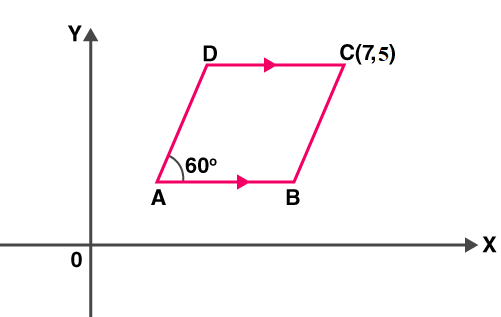Solution:

Given, ∠A = 60o and vertex C = (7, 5)

As, ABCD is a parallelogram, we have

∠A + ∠B = 180o [corresponding angles]

∠B = 180o – 60o = 120o

Slope of BC = tan 120o = tan (90o + 30o) = cot 30o = √3

So, the equation of line BC is given by

y – y1 = m(x – x1)

y – 5 = √3 (x – 7)

y – 5 = √3x – 7√3

y = √3x + 5 – 7√3

As, CD || AB and AB || x-axis

Slope of CD = Slope of AB = 0 [As slope of x-axis is zero]

So, the equation of the line CD is given by

y – y1 = m(x – x1)

y – 5 = 0(x – 7)

y = 5

11. Find the equation of the straight line passing through origin and the point of intersection of the lines x + 2y = 7 and x – y = 4.

Solution:

The given line equations are:

x + 2y = 7 ….(1)

x – y = 4 ….(2)

On solving the above line equations, we can find the point of intersection of the two lines.

So, subtracting (2) from (1), we get

3y = 3

y = 1

Now,

x = 4 + y = 4 + 1 = 5 [From (2)]

It’s given that,

The required line passes through (0, 0) and (5, 1).

The slope of the line = (1 – 0)/ (5 – 0) = 1/5

Hence, the required equation of the line is given by

y – y1 = m(x – x1)

y – 0 = 1/5(x – 0)

5y = x

x – 5y = 0